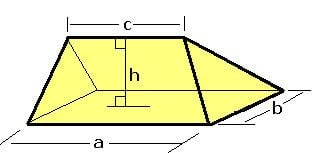# Wedge (geometry)

Updated on
Covid-19In solid geometry, a wedge is a polyhedron defined by two triangles and three trapezoid faces. A wedge has five faces, nine edges, and six vertices.

## Contents

A wedge is a subclass of the prismatoids with the base and opposite ridge in two parallel planes.

A wedge can also be classified as a digonal cupola.

Comparisons:

• A wedge is a parallelepiped where a face has collapsed into a line.
• A quadrilaterally-based pyramid is a wedge in which one of the edges between two trapezoid faces has collapsed into a point.
• ## Volume

For a rectangle based wedge, the volume is

V = b h ( a 3 + c 6 ) ,

where the base rectangle is a by b, c is the apex edge length parallel to a, and h the height from the base rectangle to the apex edge.

## Examples

Wedges can be created from decomposition of other polyhedra. For instance, the dodecahedron can be divided into a central cube with 6 wedges covering the cube faces. The orientations of the wedges are such that the triangle and trapezoid faces can connect and form a regular pentagon.

A triangular prism is a special case wedge with the two triangle faces being translationally congruent.

Two obtuse wedges can be formed by bisecting a regular tetrahedron on a plane parallel to two opposite edges.

Topics